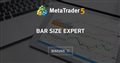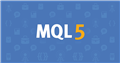# Detect volatility

I would like my EA to have separate TP and SL for when it's volatile and when it's calm.

How should I detect it? (I don't want to use an indicator)

Will taking the average size of all the bars in the last week be enough? Anything above it would be considered volatile?

Is there a quick way to get an average size of X bars or do I have to loop through all of them?

I think I found my answer

https://www.mql5.com/en/forum/102326Bar size expert
• 2007.01.22
• www.mql5.com
Hello experts, I´ve been trying to develop my own expert but it is harder than I thought...

First week of month is volatile and it is from news, also you should consider trading hours, night hours are very calm.

you also should consider using ATR(100)

I use ATR or standard deviation to measure market volatility. If you don't want to use any kind of indicators, consider using mathematical calculation.

### Calculation

1. Calculate the SMA for Period n
2. Subtract the SMA value from step one from the Close for each of the past n Periods and square them
3. Sum the squares of the differences and divide by n
4. Calculate the square root of the result from step three

Standard deviation = Sqrt [(Sum the ((Close for each of the past n Periods – n Period SMA for current bar)^2))/ n]

```  // calculate simple moving average
double calculateSma(int period){
double closePrice = 0;
double sma;
for(int i=1; i<period; i++){
closePrice = closePrice + Close[i];
}
sma = (closePrice + Bid)/period;
return sma;
}
// calculate standard deviation
double calculateStd(int period){
double sum = 0;
double std = 0;
for(int i=1; i<period; i++){
sum = sum + (MathPow((Close[i] - calculateSma(period)), 2));
}
std = MathSqrt(sum/period);
return std;
}```

The higher standard deviation means the higher volatility. You also can convert the standard deviation value to pips by multiple by Point.Documentation on MQL5: Constants, Enumerations and Structures / Objects Constants / Object Types
• www.mql5.com
Object Types - Objects Constants - Constants, Enumerations and Structures - MQL5 Reference - Reference on algorithmic/automated trading language for MetaTrader 5
Reason: